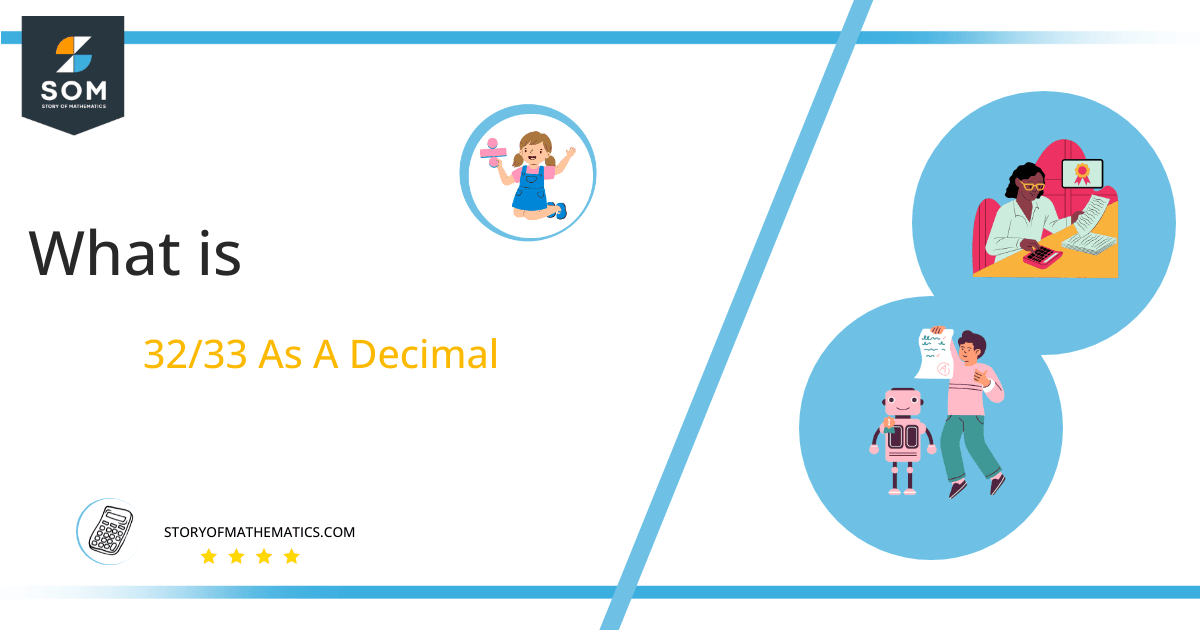# What Is 32/33 as a Decimal + Solution With Free Steps

The fraction 32/33 as a decimal is equal to 0.969.

The fraction 32/33 is a proper fraction because the value of the numerator is smaller than the value of the denominator. Its inverse is an improper fraction where the numerator is greater than the denominator.

Besides these two types, there is another type of fraction known as a mixed fraction. It is a combination of a whole number and a fraction.Here, we are more interested in the division types that result in a Decimal value, as this can be expressed as a Fraction. We see fractions as a way of showing two numbers having the operation of Division between them that result in a value that lies between two Integers.

Now, we introduce the method used to solve said fraction to decimal conversion, called Long Division, which we will discuss in detail moving forward. So, let’s go through the Solution of fraction 32/33.

## Solution

First, we convert the fraction components, i.e., the numerator and the denominator, and transform them into the division constituents, i.e., the Dividend and the Divisor, respectively.

This can be done as follows:

Dividend = 32

Divisor = 33

Now, we introduce the most important quantity in our division process: the Quotient. The value represents the Solution to our division and can be expressed as having the following relationship with the Division constituents:

Quotient = Dividend $\div$ Divisor = 32 $\div$ 33

This is when we go through the Long Division solution to our problem. Figure 1 contains the solution for fraction 32/33.Figure 1

## 32/33 Long Division Method

We start solving a problem using the Long Division Method by first taking apart the division’s components and comparing them. As we have 32 and 33, we can see how 32 is Smaller than 33, and to solve this division, we require that 32 be Bigger than 33.

This is done by multiplying the dividend by 10 and checking whether it is bigger than the divisor or not. If so, we calculate the Multiple of the divisor closest to the dividend and subtract it from the Dividend. This produces the Remainder, which we then use as the dividend later.

Now, we begin solving for our dividend 32, which after getting multiplied by 10 becomes 320.

We take this 320 and divide it by 33; this can be done as follows:

320 $\div$ 33 $\approx$ 9

Where:

33 x 9 = 297

This will lead to the generation of a Remainder equal to 320 – 297 = 23. Now this means we have to repeat the process by Converting the 23 into 230 and solving for that:

230 $\div$ 33 $\approx$ 6

Where:

33 x z2 = 198

This, therefore, produces another Remainder which is equal to 230 – 198 = 32. Now we must solve this problem to Third Decimal Place for accuracy, so we repeat the process with dividend 320.

320 $\div$ 33 $\approx$ 9

Where:

33 x 9 = 297

Finally, we have a Quotient generated after combining the three pieces of it as 0.969, with a Remainder equal to 23.Images/mathematical drawings are created with GeoGebra.